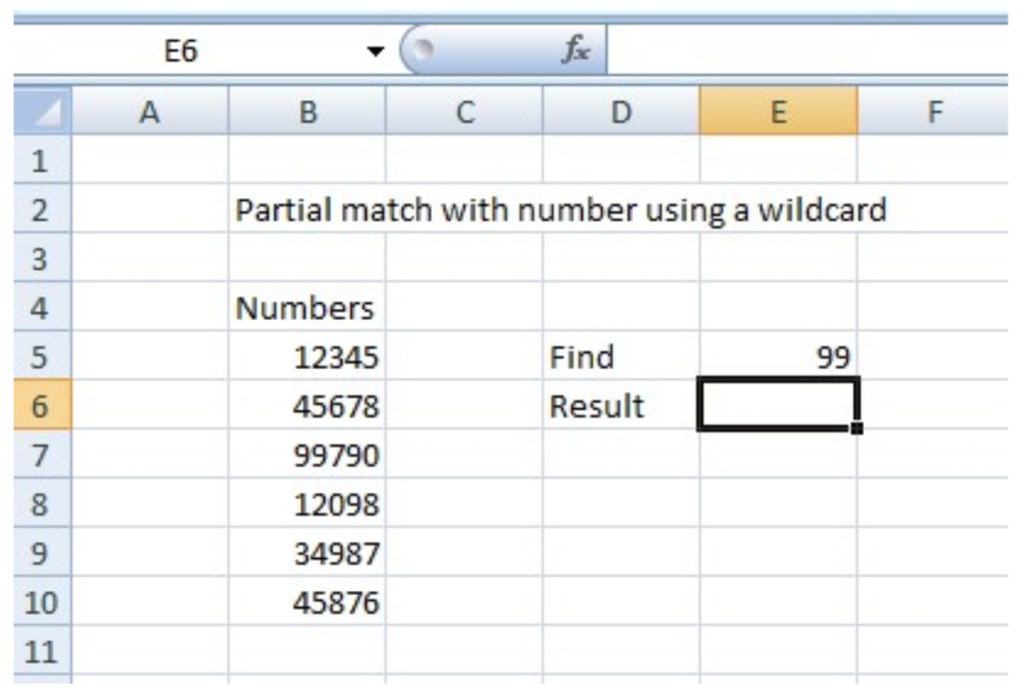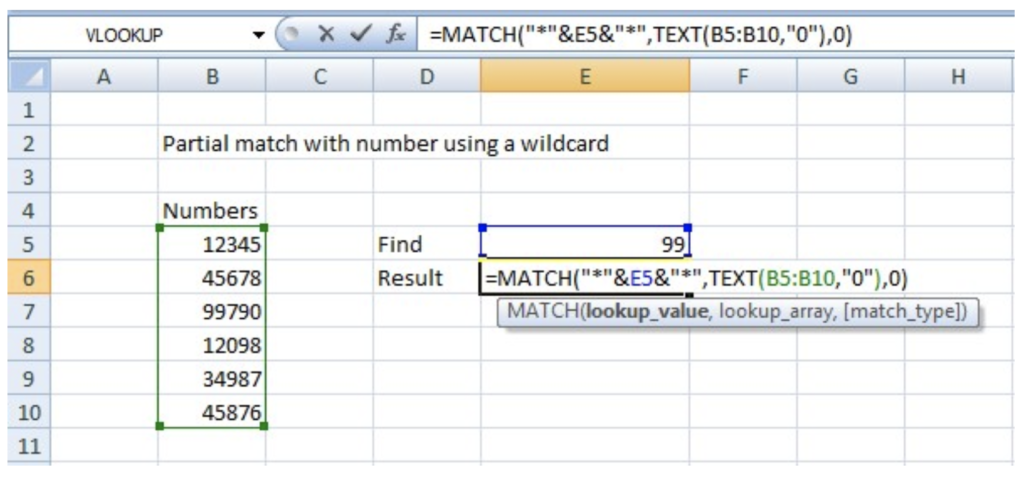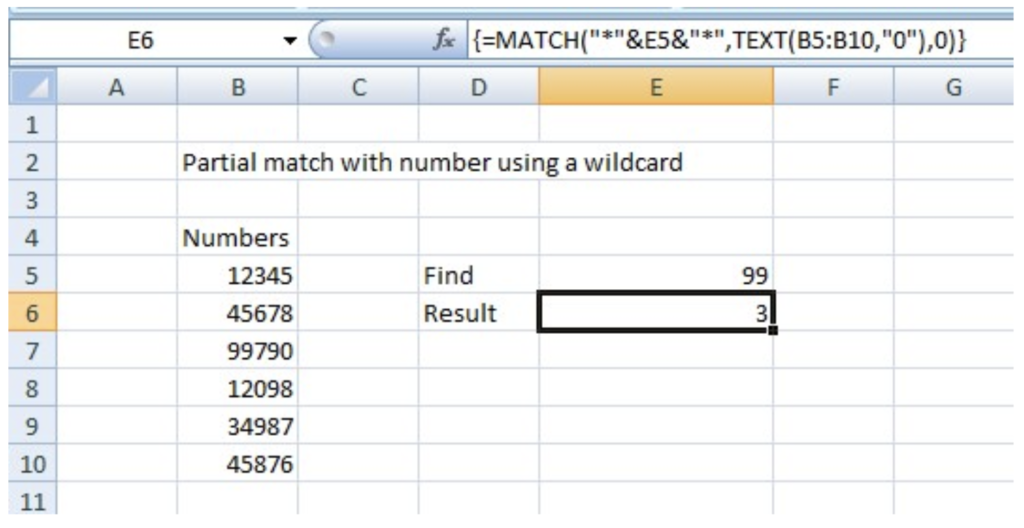Get instant live expert help with Excel or Google Sheets“My Excelchat expert helped me in less than 20 minutes, saving me what would have been 5 hours of work!”

#### Post your problem and you’ll get expert help in seconds.

Your message must be at least 40 characters
Our professional experts are available now. Your privacy is guaranteed.

# How to Find a Partial Match Against Numbers with a Wildcard in Excel

The Wildcard character is used to signify a dummy character to substitute the actual character in the text. So, ultimately you won’t need to search the actual text but can look up the partial text.

### What is a Partial match against numbers with a wildcard in Excel?

The Partial Match against numbers with the wildcard is done to employ criteria of searching the partial match of the text.

### How the Partial match against numbers with the wildcard is used in Excel

The use of the Partial match against numbers with the wildcard is used to perform the partial match against numbers. Hence, you must use the MATCH and TEXT functions for the lookup. The working of the function is explained in the Example.

Formula or Syntax using MATCH

The formula that must be used is

`{=MATCH(“*”&number&”*”,TEXT(range,“0”),0)}`

### Parameters or Arguments of a Partial match against numbers with wildcard

The arguments that must be passed in the function are:

•         Lookup_value: Required. This is the value to be matched in the array.
•         Lookup_array: Required. It is the range of cells or array reference.
•         Match_type: Optional. It is the criterion which is used for the match, specified as -1, 0, or 1.
•         Format_text: Required. It defines the number format that must be used in the lookup.

### Example:

We will consider a direct example to perform a partial match against numbers with a wildcard:

1. Construct a table of several numbers and use the array formula based on the MATCH and TEXT functions.Figure 1. Construct a table with several numbers

1. You have an option to convert the lookup range to text values if you want to perform a normal lookup with the MATCH and VLOOKUP functions. But, there is another option to use the TEXT function to convert the values within the formula.Figure 2. Insert the MATCH and TEXT function

1. Now, use the formula as `=MATCH (“*”&E5”*”, TEXT (B5: B10, ”0”), 0)` and press Control+ Shift+ Enter to apply the array formula. The TEXT function will transform the numbers into text, and the MATCH function will find the partial match. The Return will be given in the respective field as shown in the figure.Figure 3. The Return value will be given in Result Cell

### Notes on Usage of the Partial match against numbers with wildcard

The note on usage is given below:

•         The default value of the Match type is 1.
•         The match function gives the relative position of the item we are trying to find in the array.
•         The TEXT function is useful when you want to embed the numeric output of a formula and represent it in some other format inside a text.
•         The Format_text must appear in the quotation marks.

Still need some help with Excel formatting or have other questions about Excel? Connect with a live Excel expert here for some 1 on 1 help. Your first session is always free.

### Did this post not answer your question? Get a solution from connecting with the expert.Another blog reader asked this question today on Excelchat:
Solution examplesI need to add an formula into this one: =IF(\$L\$1="","",VLOOKUP(TEXT(\$L\$1,"000000"),'FRS102 BASE & GBP TB MAY 2018'!\$E:\$X,MATCH(A25,'FRS102 BASE & GBP TB MAY 2018'!\$Q\$3:\$X\$3,0)+3,0))
Solved by K. D. in 11 minsI have a pivot table in which per order (on the rows) stands how much products they ordered per size (on the columns). I want to determine which combinations of quantities of sizes people order. And I want to count these combinations.
Solved by E. W. in 60 minsI have 500 numbers in column A with aproximatly 15digits. In column B I have 5 numbers with 6 digits Can a find a formula wich can transfer all numbers from A column wich start with some 6 digitsa from B column
Solved by V. L. in 32 minsI have a sheet with 3 columns. First column is a code for records in column B (has 563 records). What I need is a formula to show me if what is in column C (has 4400 records) is in column B and if it is to take the code for that record. Example: Column A(code corresponding to column B): 12, 14, 15, 19 Column B(names): asd, adf, ade, aqw Column C(names): akd, adf, ade, anb, wgs I need something that would say, if record in column C (for example adf) is matching record in column B (adf) return the code from column A (the code corresponding to that record in column B),if not return blank.
Solved by C. J. in 8 minsIf the data in A matches the data in C, then I need the data in E to be placed in B
Solved by X. W. in 40 mins## Subscribe to Excelchat.coAnother blog reader asked this question today on Excelchat: